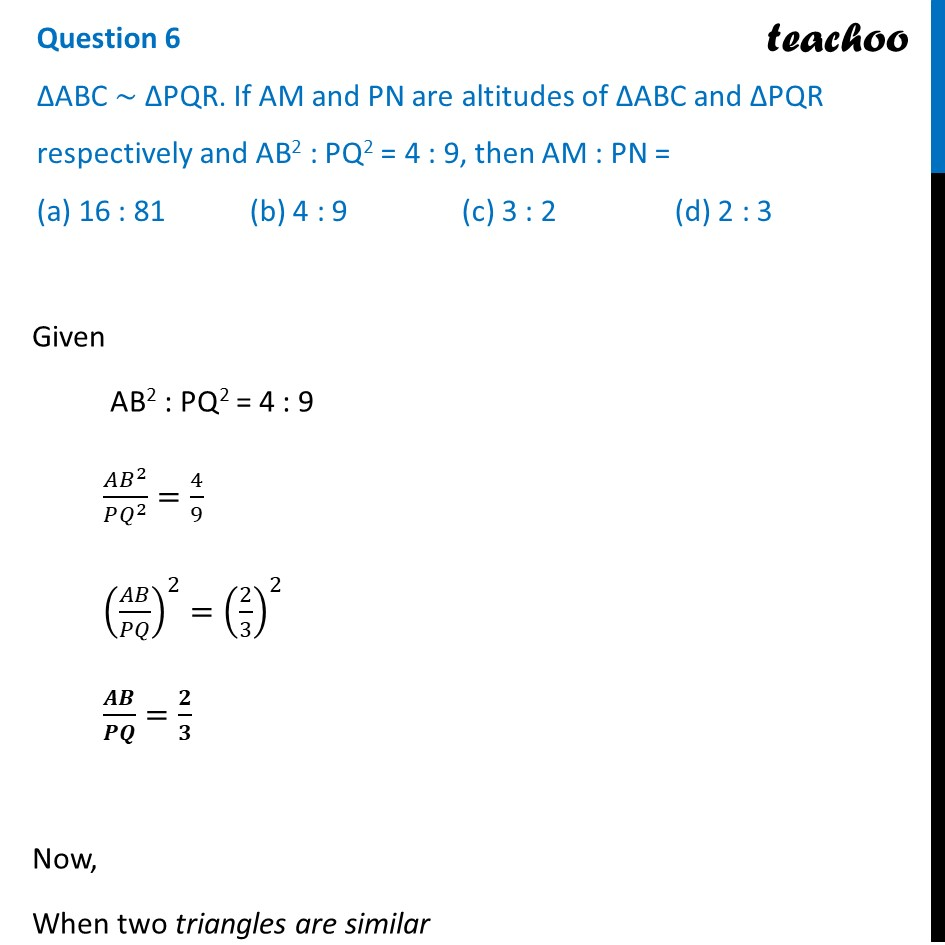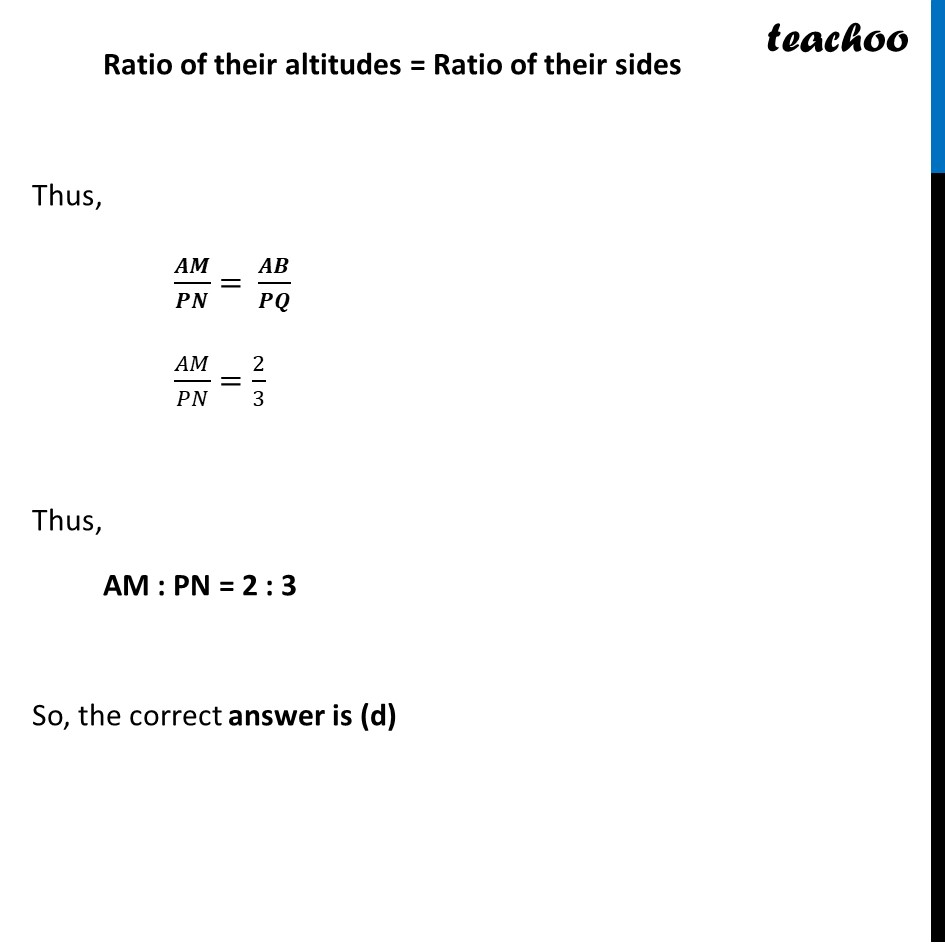CBSE Class 10 Sample Paper for 2022 Boards - Maths Standard [MCQ]

Class 10
Solutions of Sample Papers for Class 10 Boards

## (a) 16 : 81   (b) 4 : 9   (c) 3 : 2   (d) 2 : 3Get live Maths 1-on-1 Classs - Class 6 to 12

### Transcript

Question 6 ∆ABC ~ ∆PQR. If AM and PN are altitudes of ∆ABC and ∆PQR respectively and AB2 : PQ2 = 4 : 9, then AM : PN = (a) 16 : 81 (b) 4 : 9 (c) 3 : 2 (d) 2 : 3 Given AB2 : PQ2 = 4 : 9 (𝐴𝐵^2)/(𝑃𝑄^2 )=4/9 (𝐴𝐵/𝑃𝑄)^2=(2/3)^2 𝑨𝑩/𝑷𝑸=𝟐/𝟑 Now, When two triangles are similar Ratio of their altitudes = Ratio of their sides Thus, 𝑨𝑴/𝑷𝑵=" " 𝑨𝑩/𝑷𝑸 𝑨𝑩/𝑷𝑸=𝟐/𝟑 So, the correct answer is (d)